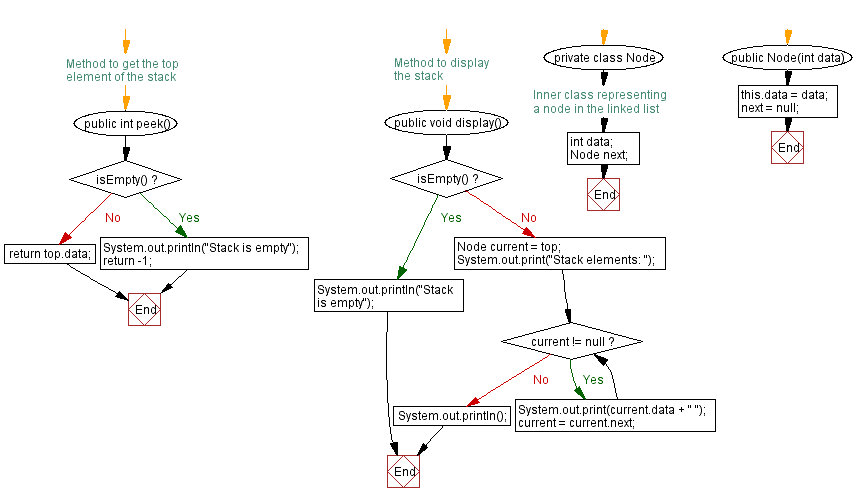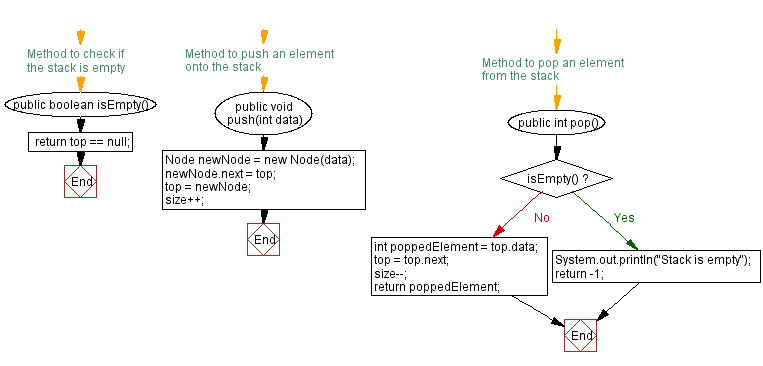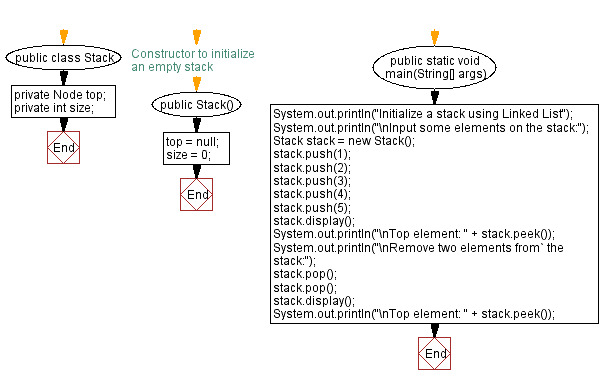# Java Exercises: Implement a stack using a linked list

## Java Stack: Exercise-29 with Solution

Write a Java program to implement a stack using a linked list.

Sample Solution:

Java Code:

``````public class Stack {

private Node top;
private int size;

// Constructor to initialize an empty stack
public Stack() {
top = null;
size = 0;
}

// Method to check if the stack is empty
public boolean isEmpty() {
}

// Method to push an element onto the stack
public void push(int data) {
Node newNode = new Node(data);
newNode.next = top;
top = newNode;
size++;
}

// Method to pop an element from the stack
public int pop() {
if (isEmpty()) {
System.out.println("Stack is empty");
return -1;
}
int poppedElement = top.data;
top = top.next;
size--;
return poppedElement;
}

// Method to get the top element of the stack
public int peek() {
if (isEmpty()) {
System.out.println("Stack is empty");
return -1;
}
}

// Method to display the stack
public void display() {
if (isEmpty()) {
System.out.println("Stack is empty");
} else {
Node current = top;
System.out.print("Stack elements: ");
while (current != null) {
System.out.print(current.data + " ");
current = current.next;
}
System.out.println();
}
}

// Inner class representing a node in the linked list
private class Node {
int data;
Node next;

public Node(int data) {
this.data = data;
next = null;
}
}

public static void main(String[] args) {
System.out.println("Initialize a stack using Linked List");
System.out.println("\nInput some elements on the stack:");
Stack stack = new Stack();
stack.push(1);
stack.push(2);
stack.push(3);
stack.push(4);
stack.push(5);
stack.display();
System.out.println("\nTop element: " + stack.peek());
System.out.println("\nRemove two elements from the stack:");
stack.pop();
stack.pop();
stack.display();
System.out.println("\nTop element: " + stack.peek());
}
}
```
```

Sample Output:

```Initialize a stack using Linked List

Input some elements on the stack:
Stack elements: 5 4 3 2 1

Top element: 5

Remove two elements from the stack:
Stack elements: 3 2 1

Top element: 3
```

Flowchart:Live Demo:

Java Code Editor:

Improve this sample solution and post your code through Disqus

Java Stack Previous: Remove elements from a stack that do not meet a condition

What is the difficulty level of this exercise?

Test your Programming skills with w3resource's quiz.

﻿